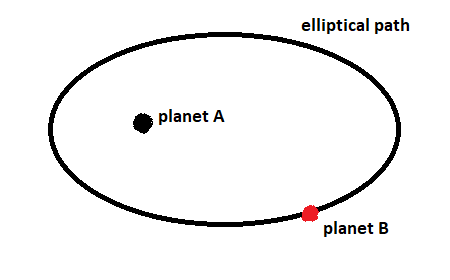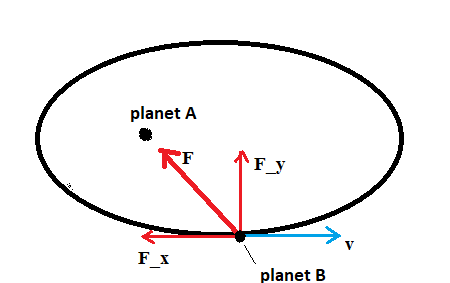# Why does the force of gravity change the speed of a satellite in an elliptical orbit? Choose the...

## Question:

Why does the force of gravity change the speed of a satellite in an elliptical orbit? Choose the correct answer

1) The force of gravity is always tangent to the satellite motion.

2) Gravity speeds up the satellite as it moves away and slows it on its return.

3) The force of gravity is always perpendicular to the satellite motion.

4) Gravity slows the satellite as it moves away and speeds it up on its return.

## Orbit:

An object is said to be in orbit if it is moving around another object in a continous curved or circular path. For example, the Earth completes its orbit around the Sun once every year.

The image below shows an example of an elliptical orbit.In circular motion, the force of gravity is always perpendicular to the planet's direction of motion. However, the gravitational force does do work on a satellite in elliptical orbit because there is a component of the force in the direction the satellite moves, as shown in the image below.The speed of planet B is going to be a maximum where its kinetic energy is maximum and its gravitational potential energy is minimum. This happens when planet B is closest to planet A.

Answer: The force of gravity change the speed of a satellite in an elliptical orbit because 4) Gravity slows the satellite as it moves away and speeds it up on its return.